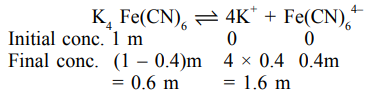# Solve this following`
Question:

A 1 molal $\mathrm{K}_{4} \mathrm{Fe}(\mathrm{CN})_{6}$ solution has a degree of dissociation of $0.4$. Its boiling point is equal to that of another solution which contains $18.1$ weight percent of a non electrolytic solute $\mathrm{A}$. The molar mass of $\mathrm{A}$ is

u. (Round off to the Nearest Integer).

[Density of water $=1.0 \mathrm{~g} \mathrm{~cm}^{-3}$ ]

Solution:Effective molality $=0.6+1.6+0.4=2.6 \mathrm{~m}$

For same boiling point, the molality of another solution should also be $2.6 \mathrm{~m}$.

Now, $18.1$ weight percent solution means $18.1 \mathrm{gm}$ solute is present in $100 \mathrm{gm}$ solution and hence, $(100-18.1=) 81.9 \mathrm{gm}$ water.

Now, $\quad 2.6=\frac{18.1 / \mathrm{M}}{81.9 / 1000}$

$\therefore$ Molar mass of solute, $\mathrm{M}=85$